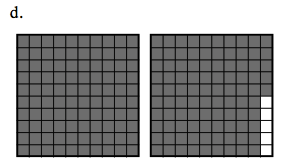### Home > MC1 > Chapter 4 > Lesson 4.3.4 > Problem4-119

4-119.
1. . For each of the representations below, write the portion in each of the forms listed. Remember that one 100-block now represents 100%.
Homework Help ✎

• Percent

• Decimal

• Fraction

• Description in words

 a.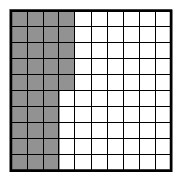b.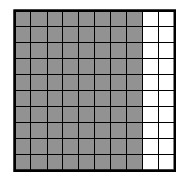c.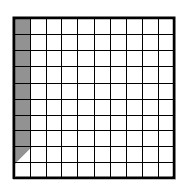d.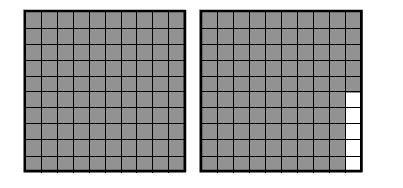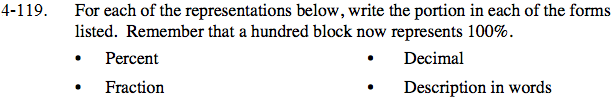If you are having trouble getting started, refer to problems MC1 4-96 and MC1 4-98 for help.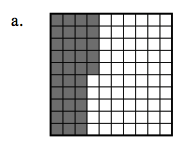3 full columns out of 10 are shaded, plus half of another column. How can you write this in the forms listed above?

Percent: 35%

$\text{Fractions: }\frac{35}{100} \text{ or }\frac{7}{20}$

Decimal: 0.35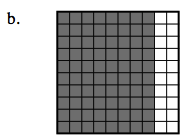This problem is similar to part (a).

Words: Thirty-five hundredths or three tenths and five hundredths or thirty-five out of one hundred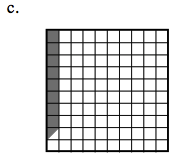To represent five and one-half hundredths,

$\text{you would write 5.5\%, }\ \frac{55}{1000},\text{ or } 0.055.$